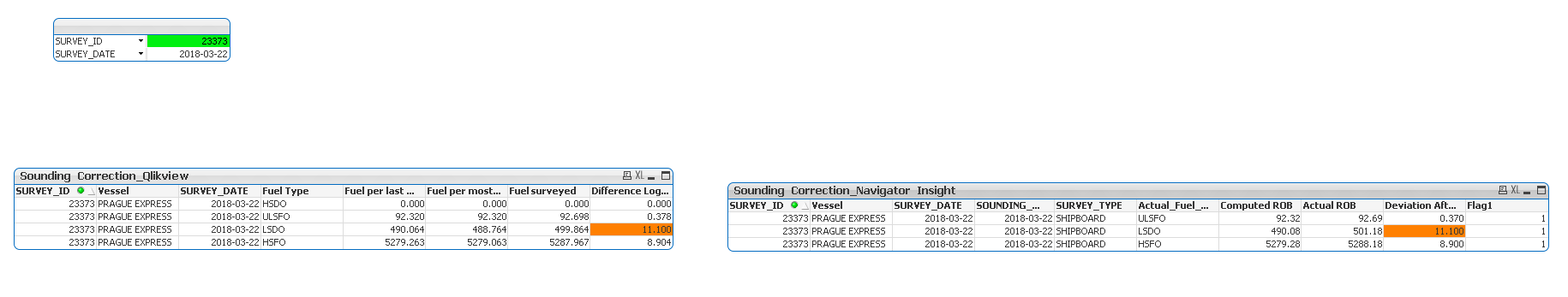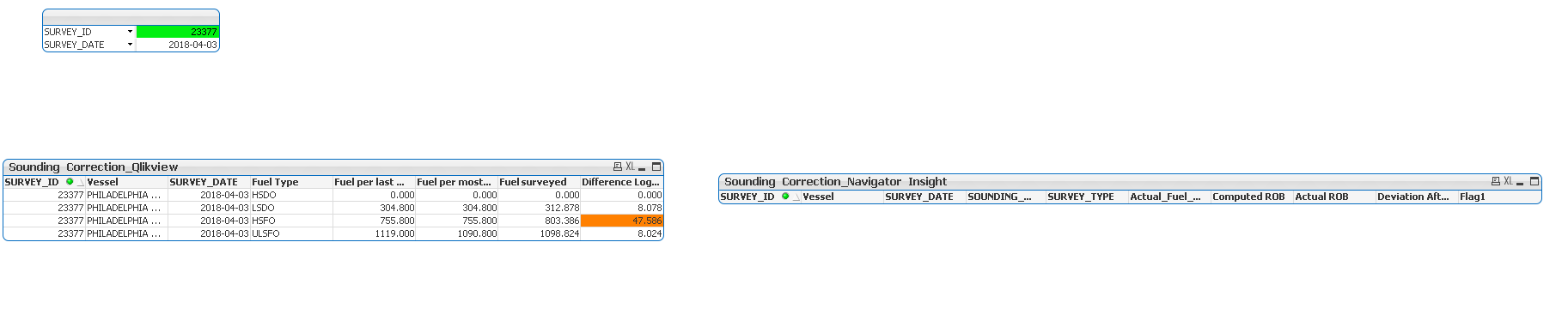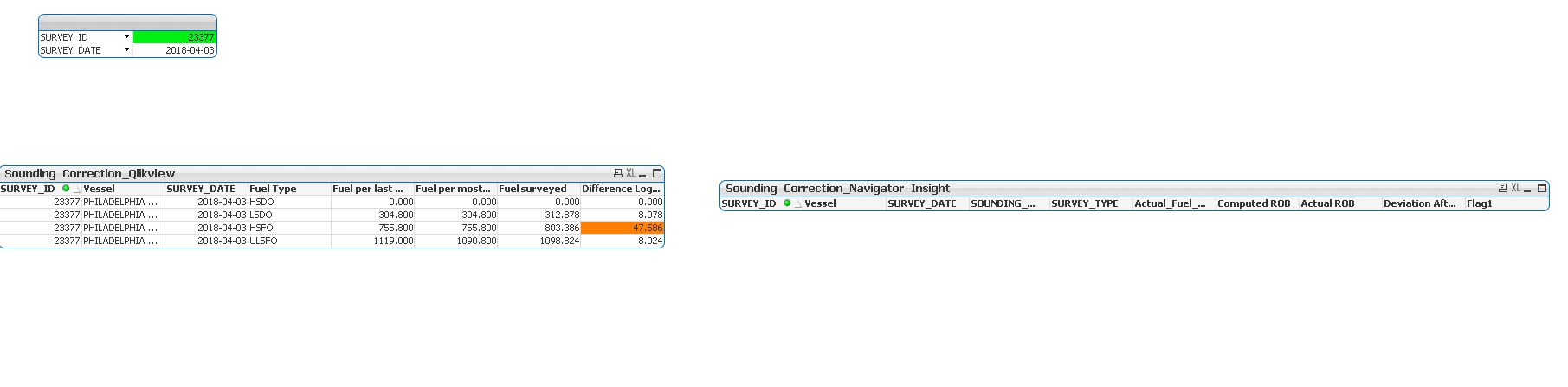# New to QlikView

Discussion board where members can get started with QlikView.

Announcements
Make your voice heard! Participate in the 2020 Wisdom of Crowds® Survey. BEGIN SURVEY
Contributor III

## How to merge 2 flag condition in same expression.

Currently I have a following syntax for the following image:If(Flag1=1,if (Match([Actual_Fuel_Type],'HSFO'), sum( total  {\$<Actual_Fuel_Type={'HSFO'}>} If([SOUNDING_START_DATE] >= [SURVEY_DATE] and [SOUNDING_START_DATE] < [SURVEY_DATE] + 7, FUEL_MASS_BEFORE_SOUNDING)/ 1000),

if (Match([Actual_Fuel_Type],'LSDO'), sum( total  {\$<Actual_Fuel_Type={'LSDO'}>}If([SOUNDING_START_DATE] >= [SURVEY_DATE] and [SOUNDING_START_DATE] < [SURVEY_DATE] + 7, FUEL_MASS_BEFORE_SOUNDING)/ 1000),

if (Match([Actual_Fuel_Type],'HSDO'), sum( total  {\$<Actual_Fuel_Type={'HSDO'}>}If([SOUNDING_START_DATE] >= [SURVEY_DATE] and [SOUNDING_START_DATE] < [SURVEY_DATE] + 7, FUEL_MASS_BEFORE_SOUNDING)/ 1000),

if (Match([Actual_Fuel_Type],'ULSFO'),sum( total  {\$<Actual_Fuel_Type={'ULSFO'}>}If([SOUNDING_START_DATE] >= [SURVEY_DATE] and [SOUNDING_START_DATE] < [SURVEY_DATE] + 7, FUEL_MASS_BEFORE_SOUNDING)/ 1000),'Unknown'

)))))

where Flag=1 is given by

If([SOUNDING_START_DATE] >= [SURVEY_DATE] and [SOUNDING_START_DATE] < [SURVEY_DATE] + 7, 1, 0)

For survey id 23377 flag 0

I also want to show a new condition that whenever the flag is 0,it will also calculate the sum of FUEL_MASS_BEFORE_SOUNDING grouped by Actual_Fuel_Type. And also show the difference between [SOUNDING_START_DATE]- [SURVEY_DATE] if its more than 7 days.Because the report shows no value for flag=0 caseIs it possible to show it in the same table.All values of flag=1 and flag=0 so that whenerver flag1 is true it calculates the computed rob and actual rob fields and when flag=0 then they also fetch the values and also show a difference in number of days between the survey date and sounding date

I have attached my qvd in this regard

2 Replies
Highlighted
Valued Contributor

## Re: How to merge 2 flag condition in same expression.

Hello, Trishita!

The data of your dashboard are scrumbled?

Highlighted
Contributor III

## Re: How to merge 2 flag condition in same expression.

I did not understand what you meant..but if flag is 0 for a specific survey id example 23377And if flag is 1 for any survey id then 23373My target is to have a report where all values will be displayed if the data is between the specific date range it will show them as computed if not within the date range then also they will show up the data and also a new column which will show that difference between survey date and sounding date if its more than 7